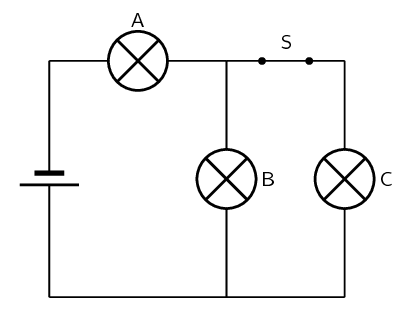Home Practice
For learners and parents For teachers and schools
Textbooks
Full catalogue
Pricing SupportLog in

We think you are located in United States. Is this correct?

# End of chapter exercises

## End of chapter exercises

Textbook Exercise 17.2

Write definitions for each of the following:

1. resistor

2. coulomb

3. voltmeter

Solution not yet available

Draw a circuit diagram which consists of the following components:

1. 2 batteries in parallel

2. an open switch

3. 2 resistors in parallel

4. an ammeter measuring total current

5. a voltmeter measuring potential difference across one of the parallel resistors

Solution not yet available

Complete the table below:

 Quantity Symbol Unit of measurement Symbol of unit e.g. Distance e.g. D e.g. kilometre e.g. $$\text{km}$$ Resistance Current Potential difference
Solution not yet available

[SC 2003/11] The emf of a battery can best be explained as the $$...$$

1. rate of energy delivered per unit current

2. rate at which charge is delivered

3. rate at which energy is delivered

4. charge per unit of energy delivered by the battery

Solution not yet available

[IEB 2002/11 HG1] Which of the following is the correct definition of the emf of a battery?

1. It is the product of current and the external resistance of the circuit.

2. It is a measure of the cell's ability to conduct an electric current.

3. It is equal to the “lost volts” in the internal resistance of the circuit.

4. It is the power supplied by the battery per unit current passing through the battery.

Solution not yet available

[IEB 2005/11 HG] Three identical light bulbs A, B and C are connected in an electric circuit as shown in the diagram below.1. How bright is bulb A compared to B and C?

2. How bright are the bulbs after switch S has been opened?

3. How do the currents in bulbs A and B change when switch S is opened?

 Current in A Current in B (a) decreases increases (b) decreases decreases (c) increases increases (d) increases decreases
1. Bulb A will be brighter than B and C as there is more current flowing through it.

2. Bulb A and B will be equally bright, but bulb C will not be lit up. (When the switch opens, no current flows through bulb C and bulbs A and B are now in series.)

3. (a). The current in A decreases, but the current in B increases.

[IEB 2004/11 HG1] When a current $$\text{I}$$is maintained in a conductor for a time of t, how many electrons with charge e pass any cross-section of the conductor per second?

1. $$It$$

2. $$\frac{It}{e}$$

3. $$Ite$$

4. $$\frac{e}{It}$$

5. $$\frac{I}{e}$$

$$\frac{I}{e}$$

If you have a circuit consisting of $$\text{4}$$ resistors of equal resistance in series and the total voltage across all of them is $$\text{17}$$ $$\text{V}$$, what is the voltage across each of them?

Solution not yet available

If you have a circuit consisting of $$\text{4}$$ resistors of equal resistance in parallel and the total voltage across all of them is $$\text{17}$$ $$\text{V}$$, what is the voltage across each of them?

Solution not yet available

In a circuit consisting of a battery and $$\text{3}$$ resistors in series, what is the voltage across the first resistor if the voltage across the battery is $$\text{12}$$ $$\text{V}$$ and the voltages across the other two resistors is $$\text{3}$$ $$\text{V}$$ and $$\text{2}$$ $$\text{V}$$ respectively?

Solution not yet available

There are $$\text{3}$$ resistors in parallel with resistances of $$\text{3}$$ $$\text{Ω}$$, $$\text{4}$$ $$\text{Ω}$$ and $$\text{11}$$ $$\text{Ω}$$. What is the total resistance of the parallel combination?

Solution not yet available

The same three resistors as above are now arranged in series, $$\text{3}$$ $$\text{Ω}$$, $$\text{4}$$ $$\text{Ω}$$ and $$\text{11}$$ $$\text{Ω}$$. What is the total resistance of the series combination?

Solution not yet available

The total resistance of two resistors in parallel is $$\text{3}$$ $$\text{Ω}$$, the one resistor has a resistance of $$\text{5}$$ $$\text{Ω}$$, what is the resistance of the other resistor?

Solution not yet available

In a series circuit there are $$\text{3}$$ resistors with voltages of $$\text{2}$$ $$\text{V}$$, $$\text{5}$$ $$\text{V}$$ and $$\text{8}$$ $$\text{V}$$, what is the voltage across the battery in the circuit?

Solution not yet available

In a parallel circuit there are $$\text{3}$$ resistors with voltages of $$\text{2}$$ $$\text{V}$$, $$\text{2}$$ $$\text{V}$$ and $$\text{2}$$ $$\text{V}$$, what is the voltage across the battery in the circuit?

Solution not yet available